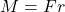Whether we realize this or not, but all of us have seen Moment in our life. Recall when you were a kid and try to sit on one end of a bench, suddenly the bench lifts. Then you move a little towards the Centre until it becomes stable.

Another case, A young man of 65Kg came and sat on the other end and wow! You just got lifted in the air for a couple of seconds.

Both these are classic examples of Moment.

Centre of Mass:  Wait. This blog is about Moment, what is COM doing here?  Well, we should know what this means before moving ahead.

Consider a perfectly regular solid ball, the one which can fit in your hand. Suppose it rests in a floor. Gravity acts on it. Right? So where exactly is that “mg” acting on the ball? Exactly at the Centre! And “Centre” would be its COM.

COM can be considered as a Centre where all the mass of that object is concentrated. So, any force which acts on COM can’t cause rotation.

Moment:  Force tends to rotate a body about a given point. Measure of this tendency is called Moment.

Mathematically, Moment is represented by M and is given by, where F is the force and r is the perpendicular distance of force from the pivot. Its unit is N.m

Since Moment has a tendency of rotation, it can be either CW (usually considered positive) or CCW (usually considered negative)

Are you thinking that these are the properties of Torque as well? They are close.

But Torque is a movement related force (E.g. Wrench) while Moment is a static force.

So, if we revisit our earlier example of bench. When the other person sits on other side of the bench, his force (due to gravity) causes the bench to rotate about that point.

More is the force; more is the tendency to rotate. Same goes with distance as well.

Food for thought: What if that person sits at Centre of bench? Will it cause any rotational tendency? If not, why?